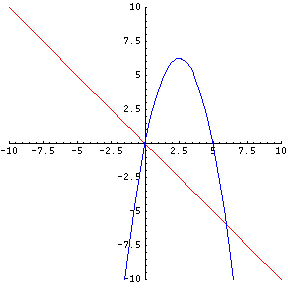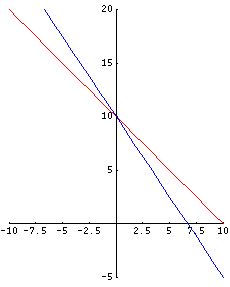# Solving a System of Equations

Systems of linear equations take place when there is more than one related math expression. For example, in $$y = 3x + 7$$, there is only one line with all the points on that line representing the solution set for the above equation.

When you are given 2 equations in the same question, and asked to solve for a unique answer, you can visualize the problem as be two lines on the same xy-plane. The following two equations are graphed on the same xy-plane:

$$y = 3x + 5$$ $$y = - x$$

The solution set to any equation is the place where BOTH equations meet on the xy-plane. This meeting place is called the Point of Intersection. If you have a linear equation and a quadratic equation on the same xy-plane, there may be TWO POINTS where the graph of each equation will meet or intersect. Here's a geometric view:Here is a sample of two equations with two unknown variables:

### Example

$$x + y = 10$$ $$3x + 2y = 20$$

There are three methods to solve our sample question.

• 1) We can solve it graphically
• 2) We can solve it algebraically
• 3) We can also solve it through algebraic elimination

I will solve the question using all 3 methods. Method 1: Solve Graphically:

To solve graphically, it is best to write BOTH equations in the slope-intercept form or in the form: $$y = mx + b$$ where m = the slope and b = the y-intercept as your first step. So, $$x + y = 10$$ becomes $$y = - x + 10$$ (slope-intercept form). Next, $$3x + 2y = 20$$ becomes $$y = -\frac{3x}{2} + 10$$ when written in slope-intercept form.

Then, graph the two lines, leading to the point of intersection. After graphing these lines, you'll find that BOTH equations meet at point (0,10). Point (0,10) means that if you plug x = 0 and y = 10 into BOTH original equations, you will find that it solves both equations. Here's what these two equations look like on the xy-plane:### Method 2: Solve algebraically

Steps:

1) Solve for eaither x or y in the first equation ($$x + y = 10$$). I will solve for y. So, $$x + y = 10$$ becomes $$y = -x + 10$$.

2) Plug the value of y (that is, -x + 10) in the second equation to find x. Our second equation was $$3x + 2y = 20$$ and, after substituting, becomes $$3x + 2(-x + 10 ) = 20$$

Next: Solve for x.

$$3x -2x + 20 = 20$$ $$x + 20 = 20$$ $$x = 0$$

3) Plug x = 0 into EITHER original equations to find the value of y. I will use our second equation.

$$3x + 2y = 20$$ $$3(0) + 2y = 20$$ $$0 + 2y = 20$$ $$y = 10$$

So, our point of intersection is once again (0,10).

### Method 3: Algebraic Elimination

This method deals with matching the variables to ELIMINATE or do away with one. Keep in mind that it is your choice which variable you want to eliminate first.

GOAL: Eliminate x and solve for y or vice-versa. Let's go back to our original equations.

In our second 3x + 2y = 20, you can eliminate 3x by multiplying -3 by EVERY term in our first equation (x + y = 10).

x + y = 10
3x + 2y = 20

-3(x) + -3(y) = -3(10)
3x + 2y = 20

-3x + -3y = -30
3x + 2y = 20

NOTICE that -3x and 3x are eliminated. See it? See why? Here's why: A negative PLUS a positive = ZERO.

We now have this:

-3y = -30
2y = 20

-3y + 2y = -30 + 20

-y = -10

y = 10.

Next: To find x, we plug y = 10 into EITHER of the original equations. By now you should see that our answer for x will be ZERO.

Here it is:

I will use x + y = 10

x + 10 = 10

x = 0.

Do you see what I see? Yes, I found the SAME point of intersection again, which is (0,10).

By Mr. Feliz
(c) 2005